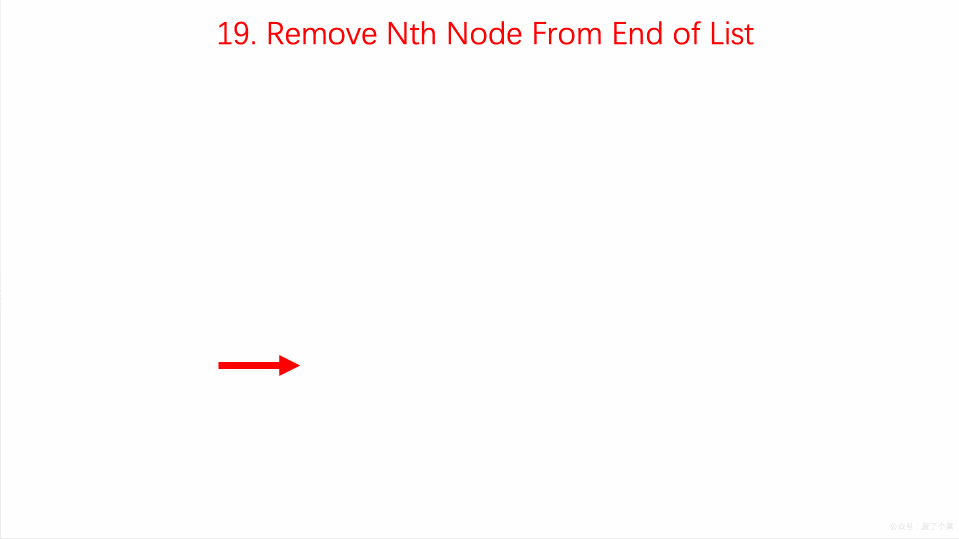By Long Luo

Here shows 3 Approaches to slove this problem: Brute Force, Stack, Slow Fast Pointers.

# Brute Froce

It’s easy to think of the way that we traverse the linked list first, from the head node to the end node, to get the length of the linked list $L$.

Then we traverse the linked list from the head node again. When the $L-n+1$ th node is traversed, it is the node we need to delete.

We can traverse $L-n+1$ nodes starting from the dummy node. When traversing to the $L-n+1$th node, its next node is the node we need to delete, so we only need to modify the pointer once to complete the deletion operation.

## Analysis

• Time Complexity: $O(n)$
• Space Complexity: $O(n)$

# Stack

We can also push all nodes on the stack one by one while traversing the linked list.

As the stack is FILO, the nth node we pop out of the stack is the node that needs to be deleted, and the node at the top of the stack is the predecessor node of the node to be deleted.

In this way, the deletion operation becomes very convenient.

## Analysis

• Time Complexity: $O(n)$
• Space Complexity: $O(n)$

# Slow Fast Pointers

1. Set $dummyHead$ to point to head node;
2. Set the two pointers $slow$ and $fast$, point to the node $dummyHead$;
3. Move $fast$ until the number of elements between $slow$ and $fast$ is $n$;
4. Move both $slow$ and $fast$ until $fast$ points to $NULL$;
5. Point the next node of $slow$ to the next node.

As the animation shows below:## Analysis

• Time Complexity: $O(n)$, $n$ is the length of the linked list.
• Space Complexity: $O(1)$

All suggestions are welcome.
If you have any query or suggestion please comment below.
Please upvote👍 if you like💗 it. Thank you:-)

Explore More Leetcode Solutions. 😉😃💗